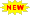Date Sheet End Semester Exam - Term23241/2324S Click Here >>Admission in PhD Program 2023-24 Click Here >>DAV University National Scholarship Test Click Here >>Department of Electrical Engineering Laboratories
• DC machine laboratory:
• Single and three phase transformers
• Different types of DC Motors
• Series/Shunt/Compound DC Generators
• AC machine laboratory:
• Load-test on three-phase Induction motor
• No-load and blocked–rotor tests on three-phase Induction motor
• Speed control of three-phase Induction motor by Kramer’s Concept
• Speed control of three-phase Induction motor by cascading of two induction motors
• Star- delta starters
• Three-phase slip–ring induction motor
• No-load and blocked–rotor test on single-phase Induction motor
• Voltage regulation of an alternator by zero power factor (ZPF.) method
• Parallel operation of three phase alternators
• Starting of synchronous motors using Auxiliary motor and Using Damper windings
• Power Electronics Laboratory:
• Operation of SCR
• Characteristics of UJT and to use UJT as relaxation oscillator
• Effect of free-wheeling diode on power factor for single phase half-wave rectifier with R-L load.
• Study of single phase full-wave, fully controlled bridge rectifier, for resistive and resistive cum inductive loads
• Microprocessor based firing control of a bridge converter
• Three phase fully controlled bridge converter
• Jones chopper
• Thyristorised speed control of a D.C. Motor
• Speed Control of induction motor using thyristors
• Study of series inverter circuit and to check its performance
• Single-phase cycloconverter
• Performance of a McMurray half-bridge inverter
• Basic Electrical and Electronics Laboratory :
• Verify Ohm’s Law, Kirchhoff’s Current Law and Kirchhoff’s Voltage Law
• Verify Thevenin’s and Norton’s theorems
• Verify Superposition theorem
• Verify Maximum Power Transfer theorem
• Study frequency response of a series R-L-C circuit and determine resonant Frequency and Q-factor for various values of R, L and C
• Frequency response of a parallel R-L-C circuit and determine resonant frequency and Q-factor for various values of R, L and C.
• Direct load test of a transformer
• Open circuit and short circuit test on transformer
• Speed control of DC motor
• Measurement of power in a three phase system by two wattmeter method
• V-I characterics of PN-junction diode
• Verify the truth table of logic gates
• Measurement and Instrumentation laboratory
• Wheatstone Bridge
• Kelvin's Bridge
• Anderson's Bridge
• Schering Bridge
• Wien’s Bridge
• Photo transistors and photo electric transducers
• Measurement of Displacement using LVDT
• Measurement of Temperature using RTD
• Light Measurement using LDR & Photo Cell
• Measurement of  Strain using Strain Gauge